#### 期刊菜单

Design of Intelligent Garbage Classification System Based on Deep Learning
DOI: 10.12677/AIRR.2022.112012, PDF, HTML, XML, 下载: 62  浏览: 179  科研立项经费支持

Abstract: At present, the front-end treatment of waste classification mainly depends on manpower, which leads to low efficiency and high cost of waste classification. In view of this situation, we designed and developed an intelligent waste classification system based on deep learning. The system automatically classifies the garbage and automatically puts it into the corresponding position through image recognition, which saves the steps of workers’ secondary sorting. At the same time, the system designs the functions of intelligent identification, control classification, GPS positioning, solar charging and intelligent smoke alarm, and develops a WeChat applet for remote monitoring to display the capacity allowance of each trash can, the amount and point of urban trash can in real time. The experimental test shows that the system has stable function and the average recognition rate of garbage can reach 93%. It has certain application value in solving the problem of garbage classification.

1. 引言

2. 系统整体结构框架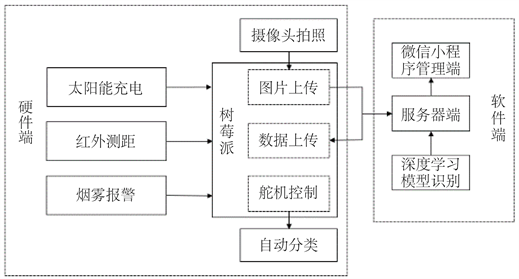Figure 1. Overall system design block diagram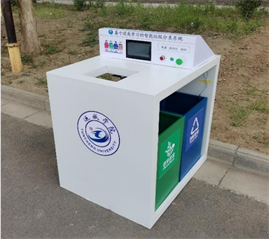Figure 2. Outdoor physical drawing of intelligent trash can

3. 系统软硬件设计

3.1. 软件开发

3.1.1. 物联网云平台

3.1.2. 微信小程序

1) 发生火情时，烟雾传感器将报警信息实时发送到小程序端自动报警，通知相关人员及时处理；

2) 通过高德地图API接入小程序，实现垃圾桶的定位功能；

3) 实时监测垃圾桶的装载余量，在垃圾桶满载时发出提醒信息，清理垃圾的相关人员实时查看数据信息，提高其工作效率 。

3.1.3. 数据集的获取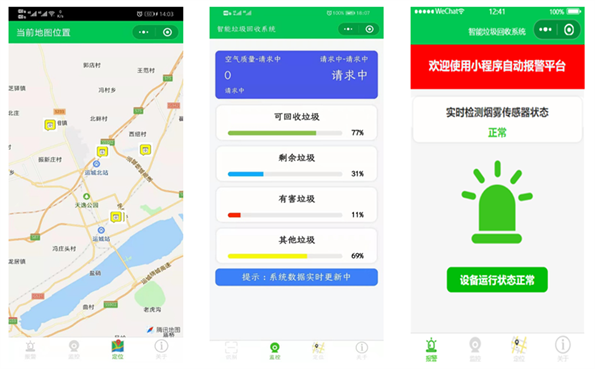Figure 3. WeChat applet management end

3.1.4. 卷积神经网络图像识别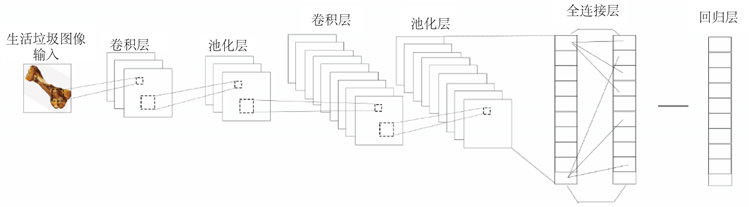Figure 4. Structure diagram of convolutional neural network

$G\left(x,y\right)=\frac{1}{2\Pi {\sigma }^{2}}{\text{e}}^{-\frac{{\left(x-{x}_{0}\right)}^{2}+{\left(y-{y}_{0}\right)}^{2}}{2{\sigma }^{2}}}$ (1)

$h\left(x,y\right)=f\ast g=\int {\int }_{-\infty }^{\infty }f\left(u,v\right)g\left(x-u,y-v\right)\text{d}u\text{d}v$ (2)

${X}_{j}^{i}=f\left(down\left({X}_{j}^{i-1}\right)+{b}_{j}^{i}\right)$ (3)

${x}^{\left(k\right)}=\frac{{x}^{k}-E\left[{x}^{\left(k\right)}\right]}{\sqrt{Var\left[{x}^{\left(k\right)}\right]}}$ (4)

3.1.5. OpenCV处理

1) 将图片的大小放缩为256 * 256；

2) 进行灰度化处理；

3) 进行高斯滤波处理；

4) 对图片进行边缘检测及二值化处理。Figure 5. Picture preprocessing process

3.1.6. 模型的训练

3.2. 硬件程序设计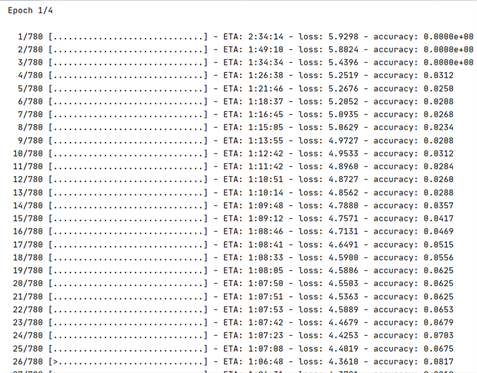Figure 6. Model training chart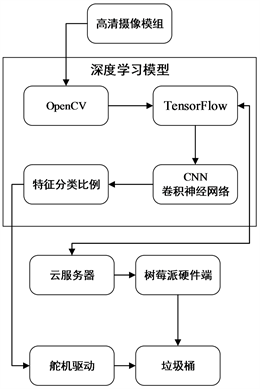Figure 7. Data information processing flow chart

3.2.1. 舵机控制程序设计

3.2.2. 太阳能充电电路设计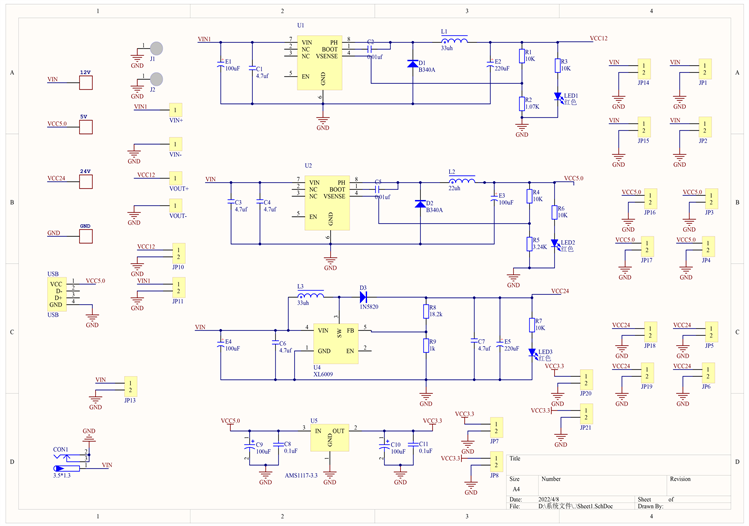Figure 8. Partial display of charging circuit diagram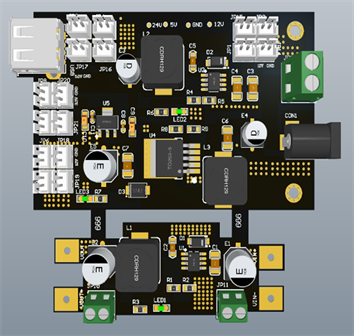Figure 9. PCB diagram of charging circuit

3.2.3. 红外测距模块

3.2.4. 烟雾报警模块

3.2.5. 树莓派摄像头拍照模块

3.3. 系统工作模式

4. MQTT通信协议

5. 系统功能验证

5.1. 实验环境

5.2. 实验结果

5.3. 结果分析Table 1. Garbage image recognition rate test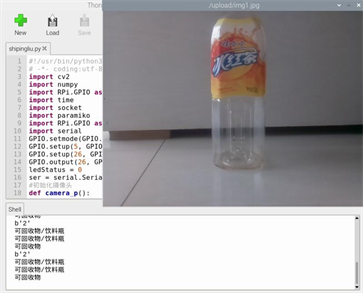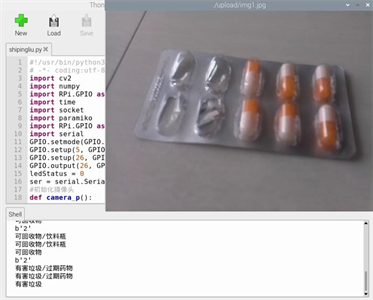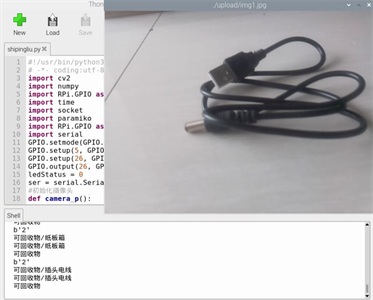Figure 10. Experimental test diagram

6. 总结

NOTES

*第一作者。

#通讯作者。

  郭昌鑫, 陈公兴. 基于深度学习的智能垃圾桶识别分类系统[J]. 科技与创新, 2020(12): 30-31+35.  张海燕, 杨治山. 基于物联网网关的智能生产线供料站的实时通讯研究[J]. 电子测试, 2021(11): 63-64+140.  黄国维. 基于深度学习的城市垃圾桶智能分类研究[D]: [硕士学位论文]. 淮南: 安徽理工大学, 2019.  高明, 高明, 张泽慧, 等. 基于新型空间注意力机制和迁移学习的垃圾图像分类算法[J]. 系统工程理论与实践, 2021, 41(2): 498-512.  李妍. 基于ResNet算法的垃圾图像识别分类研究[J]. 长江信息通信, 2021, 34(5): 25-27.  蒋超. 基于用户评论数据的线上大家电产品用户满意度影响因素研究[D]: [硕士学位论文]. 哈尔滨: 哈尔滨工程大学, 2021.  齐鑫宇, 龚劬, 李佳航, 等. 基于深度学习的垃圾图片处理与识别[J]. 电脑知识与技术, 2021, 17(9): 20-24.  胡磊. 基于深度学习的超临界机组给水流量预测及控制优化[D]: [硕士学位论文]. 太原: 山西大学, 2021.  梅吟松, 王雯雯, 陆庆文, 王涛. 放置在公共场所的可翻盖和及时清理的智能垃圾桶设计[J]. 科技创新导报, 2020, 17(10): 2.  牛岩松, 宗峰. 一种利用Raspberry搭建区块链私有链全节点的方法研究[J]. 现代计算机, 2020(19): 105-108.  杨皓文, 胡琦瑶, 李江南, 等. 基于NB-IoT的智能垃圾分类系统[J]. 物联网技术, 2020(8): 47-51.